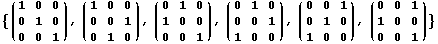This header plots the critical line of the Riemann Zeta Function.  A complete understanding wins a \$1,000,000 prize.
 . . .Main Links Orders Post Next Page Next + 10

A new version of GAP is now available.  Unless you know some Group Theory, this free program won't do much for you.  I dabble in Groups due to their strong symmetries.  My study of Fair Dice used the Sylow Theorem, which is helpful in enumerating groups of a certain size.  So then, what is a Group?

A group is an ordered pair (G, *) such that G is a set, * is an associative binary operation on G, and there exists an identity element e such that:     1.  if a is in G, then a * e = a.   2.  if a in in G, then there exists b in G such that a * b = e.

Examples of Infinite Groups: the integers where * = addition, the real numbers where * = addition, the positive real numbers > 0 where * = multiplication.  Examples of Finite Groups: A = {1, -1, i, -i} where * is multiplication, B = {0, 1, 2, 3) where * is addition modulo 4.  A subgroup is a group entirely inside another: {1, -1} is a subgroup of A, {0, 2)  is is a subgroup of B.  So far, all the groups mentioned are commutative (ab = ba for all a, b in G).  Such commutative groups are called abelian groups.  It is possible to have nonabelian groups.The above set forms a complete group under matrix multiplication.  It is equivalent to a six-sided die made by gluing two tetrahedra together. If you multiple any two of them together, you'll get one of these matrices.  Also, you could rotate the die back into a similar position in six different ways.  This is known as the Dihedral group of order 3.  If groups are new to you, remember this one thing:  groups are complete sets!  (It was this realization that allowed me to 'get it')  Other matrix groups could be made with 24 4x4 matrices, or 120 5x5 matrices, or 720 6x6 matrices.  Just put one 'one' in every row and column in all possible ways.  This makes a set of Permutation matrices.  For the 5x5 case, if you start with the vector {a,b,c,d,e}, there is a matrix multiplier which will produce {e,a,c,b,d}.  You can get any possible permutation.  These are also know as Permutation Groups.  It turns out that all groups are equivalent to some subgroup of a permutation group.  Thus, all groups can be represented with matrices.

If you find groups interesting, you can find much more in the CRC Encyclopedia of Mathematics, and Mathematical Atlas.  The largest theorem in mathematics is the ENORMOUS THEOREM, and concerns the classification of finite simple groups.  Hundreds of mathematicans worked on this theorem for many years.

Operations on Rubik's Cube can be considered a finite group.  The makers of GAP have written an analysis of Rubik's Cube from a Group Theory perspective.  With GAP, one can show that Rubik's Cube has 43,252,003,274,489,856,000 positions.

If you've played a computer game lately, you've encountered three-dimensional rotations.  These are done with 3x3 matrices.  The set of all of rotations of the sphere is a group, known as the Special Orthogonal Group -- SO(3).  All the members of this infinite set are 3x3 matrices.  Again, it is a complete set.  If you rotate a sphere one way, then another, you wind up with a different rotation of the sphere.  My Dice page shows that their are finite subgroups of SO(3).  Other than the Dihedral groups (glue two pyramids together), there are only three:  T, O, and I -- the Tetrahedral, Octahedral, and Icosahedral groups.

I have several good books about Group Theory.  Applications of Finite Groups by J. S. Lomont discusses crystals in detail, and provides generating matrices for all 32 crystal point groups. Geometry and Symmetry by Paul B. Yale.  Group Theory by W. R. Scott.  These are all difficult books, so be forewarned.